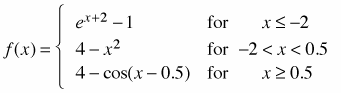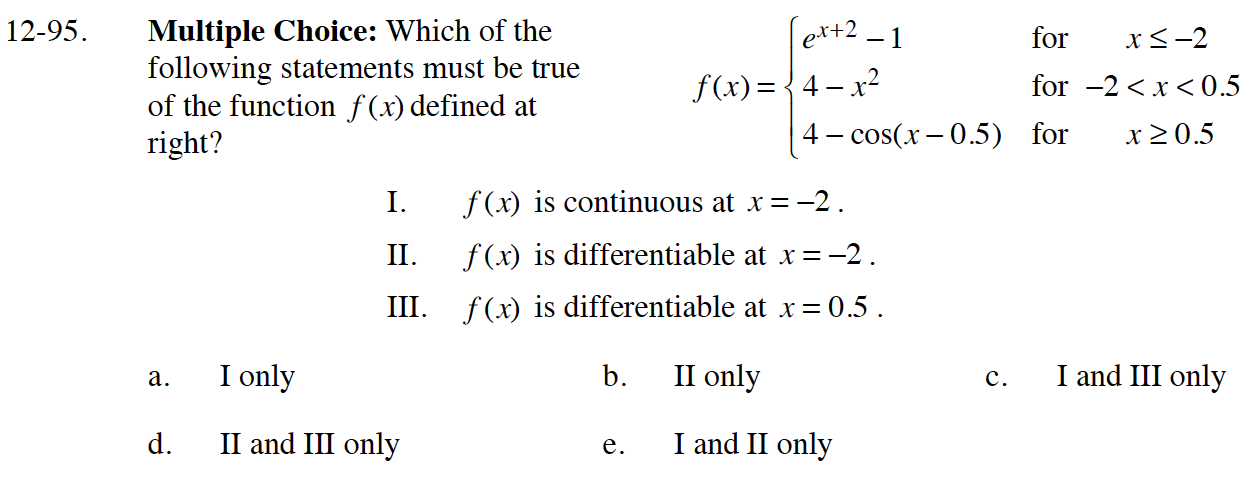### Home > CALC > Chapter 12 > Lesson 12.2.3 > Problem12-95

12-95.
1. Multiple Choice: Which of the following statements must be true of the function f(x) defined below? Homework Help ✎1. f(x) is continuous at x = −2.

2. f(x) is differentiable at x = −2.

3. f(x) is differentiable at x = 0.5 .

1. I only

2. II only

3. I and III only

4. II and III only

5. I and II onlyTherefore f is continuous at x = –2.

Therefore f is not differentiable at x = –2.